• 这是我用Matlab写的，用维纳滤波处理运动模糊，请高手指点一下，希望对大家有帮助
• matlab运动模糊图像复原matlab运动模糊图像复原matlab运动模糊图像复原matlab运动模糊图像复原matlab运动模糊图像复原
• 由于这段时间在做一个图像处理与三维重建的项目，其中数字图像处理的知识是必不可少的，学习matlab也有一段时间了，所以现在抽点时间将这段时间所学的东西做个小总结，加深理解的同时也方便以后有需要时可以查看一下...
        由于这段时间在做一个图像处理与三维重建的项目，其中数字图像处理的知识是必不可少的，学习matlab也有一段时间了，所以现在抽点时间将这段时间所学的东西做个小总结，加深理解的同时也方便以后有需要时可以查看一下。
图像运算是图像处理中常用的处理方法，它以图像为单位进行操作，运算的结果是一副新的图像，常常用于图像的高级处理（如图像分割，目标的检测和识别等）的前期处理。具体的图像运算包括点运算，代数运算，几何运算和邻域运算。点运算常用于改变图像的灰度范围及分布，从而改善图像的效果，代数运算常用于医学图像的处理以及图像误差检测，几何运算在图像配准，校正等方面有重要用途，邻域运算主要用在图像滤波和形态学运算方面。
在Matlab中，数字图像的数据是以矩阵形式存放的，矩阵的每一个元素值对应着一个像素点的像素值，这样一来，对图像的运算就相当于对数据矩阵进行运算。下面用matlab的具体图像运算实例进行介绍：

图像的（线性）点运算：
在图像处理中，点运算是一种简单而又很重要的技术，对于一副输入图像，若输出图像的每个像素点的灰度值由输入像素点决定，则这样的图像变换就成为图像的点运算。
figure(1);                    %图像显示窗口
imshow(A);                %输出图像A
B = A +50;                %A图像的灰度值增加50，成为新的图像B
figure(2);
imshow(B);           %输出图像B
C= 1.5 * A;             %A图像的对比度增强，成为新的图像C
figure(3);
imshow(C);            %输出图像C
D = 0.8 * A;            %图像对比度减弱，成为新的图像D
figure(4);
imshow(D);             %输出图像D
E = -double(A) + 255;      %对图像A进行求补，但要注意先把A的类型转化为double型，实际是E = -1 * A + 255;
figure(5);
imshow(uint8(E));                   %输出时注意将图像转为原来的 uint8 类型
图像的代数运算：
图像的代数运算是指对两幅或两幅以上的输入图像进行加减乘除四则运算，它在图像处理中有着广泛的应用，加法运算可以用来降低图像中的随机噪声，减法运算可以用来减去背景，运动背景，进行梯度幅度运算，乘法运算通常用来进行掩模运算，除法运算可以用来归一化。
图像加法：
图像减法：

C  = imsubtract(A,B);            %将A，B两幅图像进行相减得到新的图像C
图像乘法：

C  = immultiply(A,B);            %将A，B两幅图像进行相乘得到新的图像C
图像除法：

C  = imdivide(A,B);            %将A，B两幅图像进行相除得到新的图像C

图像的几何运算：
为了达到某种视觉效果，变换输入图像的像素位置，通过把输入图像的像素位置映射到一个新的位置达到改变原图像的显示效果的目的，这一过程成为图像的几何运算，图像的几何运算主要是指对图像进行几何校正，空间变换（缩放，旋转，仿射变换）等运算过程。

图像的缩放实际上是通过对图像进行插值来实现的，也就是通过增加或减少图像矩阵的行列数来实现对图像的放大和缩小操作，一般常用的插值方法有四种：分别是最近邻插值（nearest），线性插值（linear)，三次样条插值（spline），和立方插值（cubic）。
matlab中实现插值的函数是interp2，其语法格式为：
A = interp2(B, X, Y,method);                   %其中B为原图像，X 和Y为图像的新的行数和列数，method为上面说的四种插值方法
A = interp2(B, ntime, method);              %其中B为原图像，ntime为缩放倍数，method为上面说的四种插值方法
A = interp2(B ,200,300,' nearest ' );           %采用最近邻插值法将图像B转换为 [200,300] 的新图像A
C = interp2(B , 2,' linear');                             %采用线性插值法将图像B增大2倍，成为新图像C
D = interp2(B , 300,500,' spline' );             % 采用三次样条法 将图像B转换为 [300,500] 的新图像D
E  = interp2(B, 0.8,'cubic');                         % 采用立方插值法将图像B 缩小为0.8倍的新图像E

图像的邻域运算：
邻域运算主要作用是对图像进行空域滤波，比如平滑，中值滤波以及边缘检测等，此外，邻域运算还可以对结构图像进行细化。

平滑： 平滑的目的是消除或尽量减少噪声，改善图像的质量。在matlab中用imfilter函数进行平滑操作，B  = imfilter(A, h); 其中A为原图像，h表示卷积核。
h = ones(3,3)/5;              %制定卷积核
h(1,1) = 0;
h (1,3) =0;
h(3,1) = 0;
h(1,3) = 0;
B  = imfilter(A,h);               %平滑处理
figure(1);
imshow(B);
中值滤波：
中值滤波用一个含有奇数点的滑动窗口，将邻域的像素按灰度级排序，取其中间值为输出像素。它的优点在于能够在抑制随机噪声的同时不使边缘模糊，但对于线，尖顶等细节多的图像不宜采用中值滤波。
B = medfilt(A);                  %对图像A进行中值滤波
figure(1);
imshow(B);
终于总结好了matlab的图像运算，主要是点运算，代数运算，几何运算和邻域运算，这四种方法涵盖了大部分数字图像处理的常用的手段，是数字图像处理的基础内容，但是往往基础的东西便是最重要的东西。~~


展开全文图像处理 matlab
• 《数字图像处理-MATLAB运动模糊图像复原 图像复原技术也常被称为图像恢复技术图像复原技术能够去除或减轻在获取数字图像过程中发生的图像质量下降（退化）问题，从而使图像尽可能地接近于真实场景
• （注：本文代码大部分可从《数字图像处理 第三版》中找到）使用软件：MATLAB R2018a学习前提：了解matlab的GUI界面的每个按钮参考资料：《数字图像处理 第三版》，CSDN博客使用初音图片P站画师uid：1589657。...
前言：本文类似于学习笔记，所以有疑问或者有什么宝贵的建议欢迎在下方留言。（注：本文代码大部分可从《数字图像处理 第三版》中找到）

使用软件：MATLAB R2018a

参考资料：《数字图像处理 第三版》，CSDN博客

使用初音图片P站画师uid：1589657。

最终实现效果：（下图）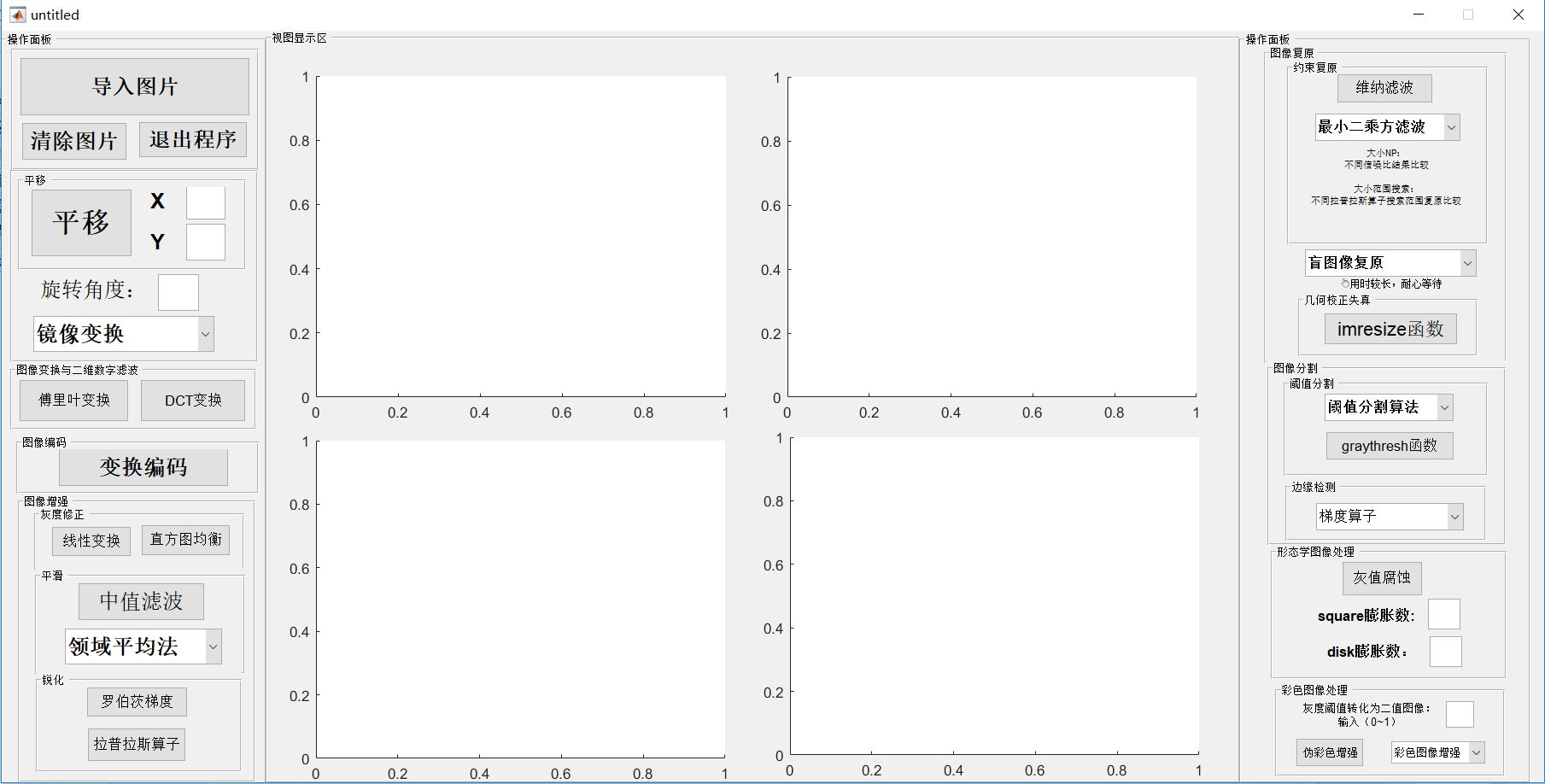正文内容

打开matlab后输入guide后弹出选择界面，新建一个GUI界面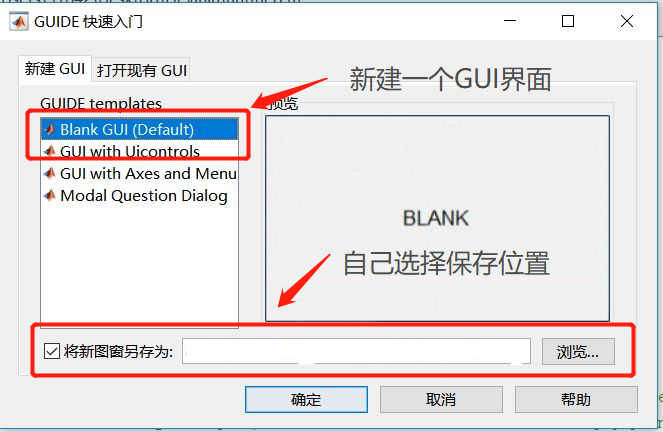在左边选择自己需要按钮在界面中展出后，右键该按钮选择查看回调→Callback打开后会自动跳转到该按钮的代码块，只要在定位到的function pushbutton1_Callback代码下写要实现的功能就可以了。

下面直接展示相关代码功能：

（1）导入图片

[file path]=uigetfile('*.bmp;*.jpg;*.png','请选择一幅图像');
if file==0 warndlg('您得输入一幅图像');
%警告对话框提示输入合法图像文件
else
axes(handles.axes1);
imshow(I);title('原图像');
handles.I=I;
end
%Update handles structure
guidata(hObject,handles);

（2）清除图片和文本框内容

%清除视图图片
cla(handles.axes2,'reset');  %handles.axes2为显示图片窗口，reset即清除
% 重置清空动态txt的文字
set(handles.edit1,'string','')；  %handles.edit1为要清除文字的文本框（双击文本框可以看见tag）

（3）关闭程序

close

（4）平移（用文本框实现输入数字进行平移图像）

①先创建一个可编辑文本框（输入水平平移的位移），然后给他添加代码

%获取text文本框的数字，竖直平移X的数值
global x;%定义一个x的全局变量
x=str2num(get(hObject,'String'));

②先创建一个可编辑文本框（输入竖直平移的位移），然后给他添加代码

%获取text文本框的数字，竖直平移Y的数值
global y;
y=str2num(get(hObject,'String'));

③创建一个button，给他添加代码

global y;
global x;
I=handles.I;
axes(handles.axes2);
se=translate(strel(1),[x y]);
j=imdilate(I,se);
axes(handles.axes2);
imshow(j);title('竖直平移后图像');

在X和Y的文本框中输入数值，点击button后就能使图像平移了（如输入X：260；Y：90）（5）旋转

%图像的旋转
I=handles.I;
a=str2num(get(hObject,'String'));
J1=imrotate(I, a);   %设置旋转角度，实现旋转并显示
axes(handles.axes2);
imshow(J1);title('旋转后图像');
guidata(hObject,handles);（6）镜像

%用下拉列表实现水平镜像，垂直镜像，水平垂直镜像
switch get(hObject,'value')   %实现下拉列表需要写改语法
case 1

case 2
I=handles.I;
J1=flipdim(I,2);%原图像的水平镜像
axes(handles.axes2);
imshow(J1);title('水平镜像');
guidata(hObject,handles);
case 3
I=handles.I;
J2=flipdim(I,1);%原图像的垂直镜像
axes(handles.axes2);
imshow(J2);title('垂直镜像');
guidata(hObject,handles);
case 4
I=handles.I;
J3=flipdim(I,1);%原图像的水平垂直镜像
J4=flipdim(J3,2);
axes(handles.axes2);
imshow(J4);title('水平垂直镜像');
guidata(hObject,handles);
end下面就不把全部代码都写出来了，只挑选一部分出来（上面代码几乎能实现每个按钮的用法）

（7）DCT变换

%DCT变换（P58）
I=handles.I;
J=rgb2gray(I);%将图片转变为灰色图像
axes(handles.axes2);
imshow(J);title('原灰图像');
K=dct2(J);%对图像做DCT变换
axes(handles.axes3);
imshow(log(abs(K))+1,[0,10]);title('DCT变换结果');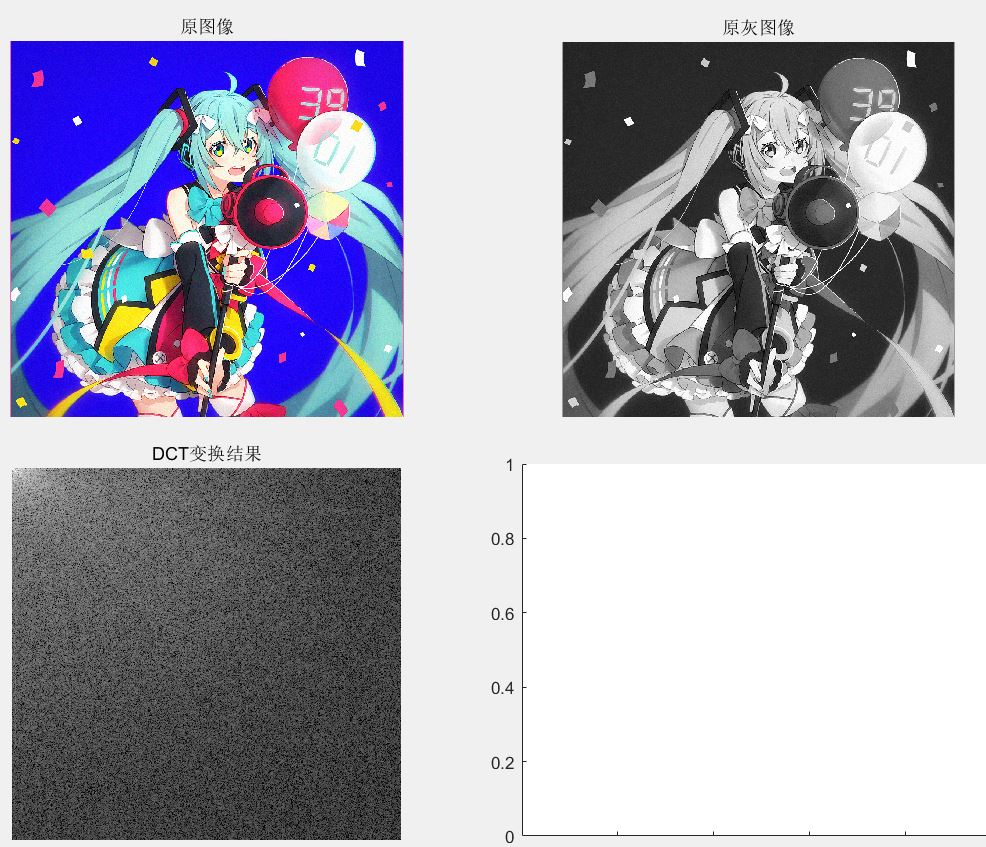（8）对受椒盐噪声污染的图像采用中值滤波去噪

I=handles.I;
I=rgb2gray(I);%转化为灰度图像
J=imnoise(I,'salt & pepper',0.04);%对图像增加椒盐噪声，强度为0.04
axes(handles.axes2);    %显示在axex2框中
imshow(J); title('受椒盐噪声污染图片');
K=medfilt2(J);          %二维中值滤波
axes(handles.axes3);
imshow(K);title('二维中值滤波处理后的图片');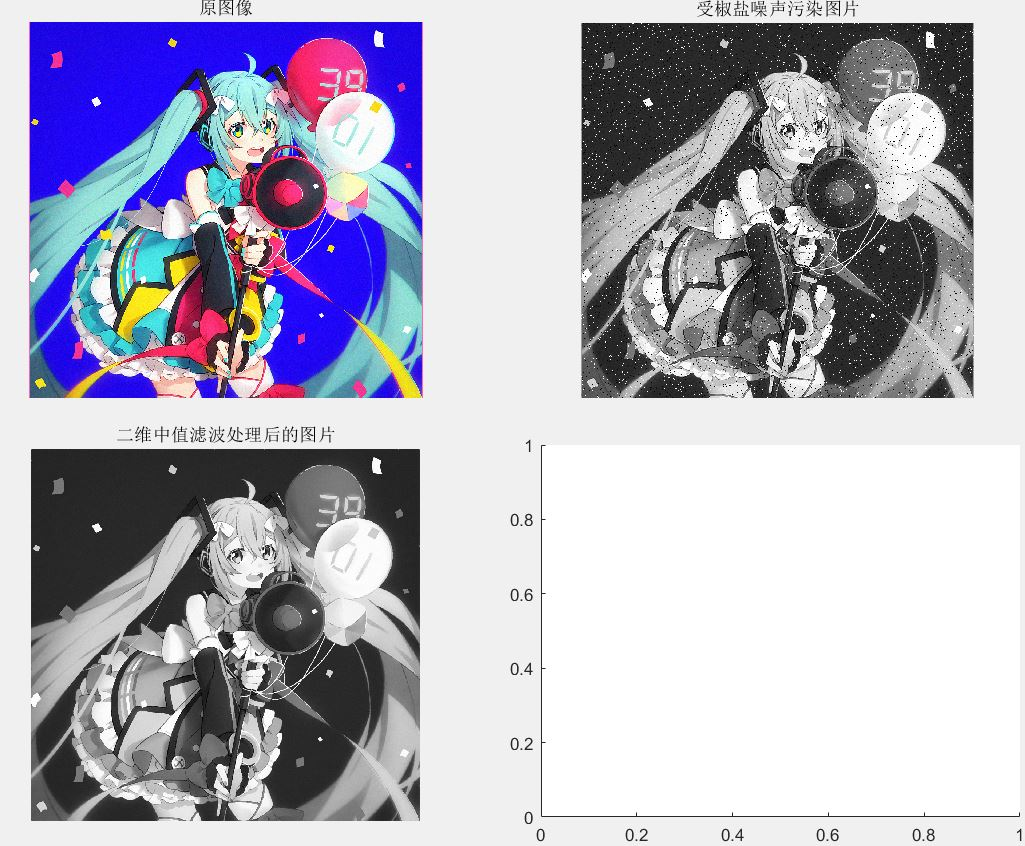（9）彩色图像增强（分别使用RGB和HSV方法）——用下拉列表实现

switch get(hObject,'value')
case 1

case 2
RGB=handles.I;
R=RGB(:,:,1);
G=RGB(:,:,2);
B=RGB(:,:,3);
R1=histeq(R);
G1=histeq(G);
B1=histeq(B);
RGB1=cat(3,R1,G1,B1);
axes(handles.axes2);    %显示在axes2框中
imshow(RGB1);title('RGB增强');
case 3
RGB=handles.I;
R=RGB(:,:,1);
G=RGB(:,:,2);
B=RGB(:,:,3);
R1=histeq(R);
G1=histeq(G);
B1=histeq(B);
RGB1=cat(3,R1,G1,B1);
[H,S,V]=rgb2hsv(R,G,B);
V=histeq(V);
[R2,G2,B2]=hsv2rgb(H,S,V);
RGB2=cat(3,R2,G2,B2);
axes(handles.axes2);    %显示在axes2框中
imshow(RGB2,[]);title('HSV增强');
C=double(RGB1)./255-RGB2;
axes(handles.axes3);    %显示在axes3框中
imshow(C);title('差值图像');
end

RGB增强:HVS增强：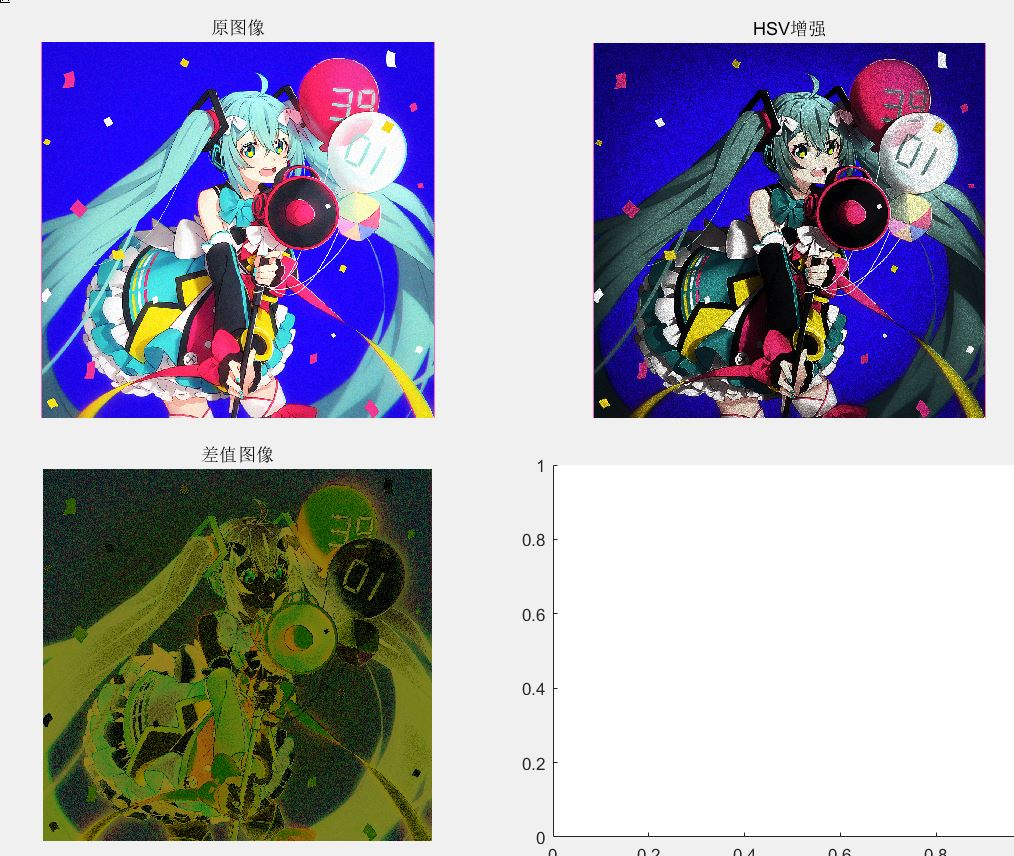（10）彩色图像锐化

代码引用了：点击打开链接

I=handles.I;
fb=tofloat(I);  	%将图像转化为浮点型
lapmask=[1 1 1;1 -8 1;1 1 1]; 	%拉普拉斯滤波模板
axes(handles.axes2);
imshow(fen);title('拉普拉斯锐化');
function [out,revertclass] = tofloat(inputimage)
%Copy the book of Gonzales
identify = @(x) x;
tosingle = @im2single;
table = {'uint8',tosingle,@im2uint8
'uint16',tosingle,@im2uint16
'logical',tosingle,@logical
'double',identify,identify
'single',identify,identify};
classIndex = find(strcmp(class(inputimage),table(:,1)));
if isempty(classIndex)
error('不支持的图像类型');
end
out = table{classIndex,2}(inputimage);
revertclass = table{classIndex,3};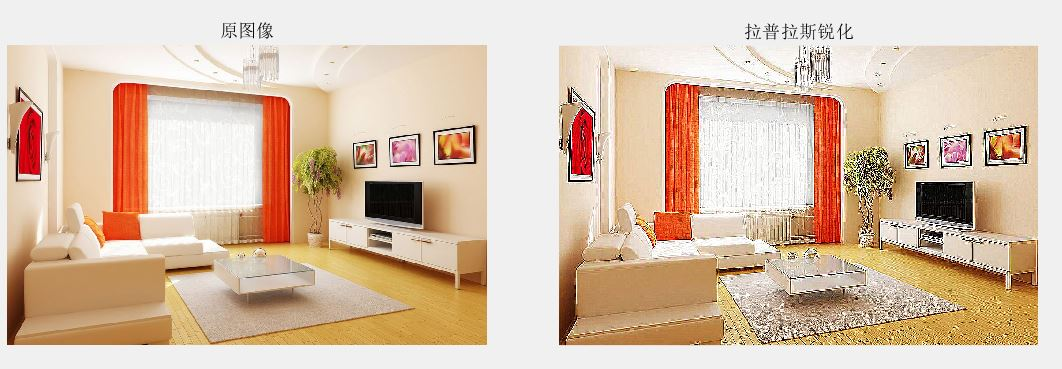（10）彩色图像复原

%维纳滤波复原图像
I=handles.I;
len=28;
theta=14;
PSF=fspecial('motion',len,theta);
blurred=imfilter(I,PSF,'circular','conv');%读入无噪声模糊图像，并命名blurred
len=28;
theta=14;
wnrl=deconvwnr(blurred,PSF,0.04);%维纳滤波复原图像
axes(handles.axes2);
imshow(blurred);title('由运动形成模糊图像');%显示模糊图像
axes(handles.axes3);
imshow(wnrl);title('维纳滤波复原图像');%显示复原图像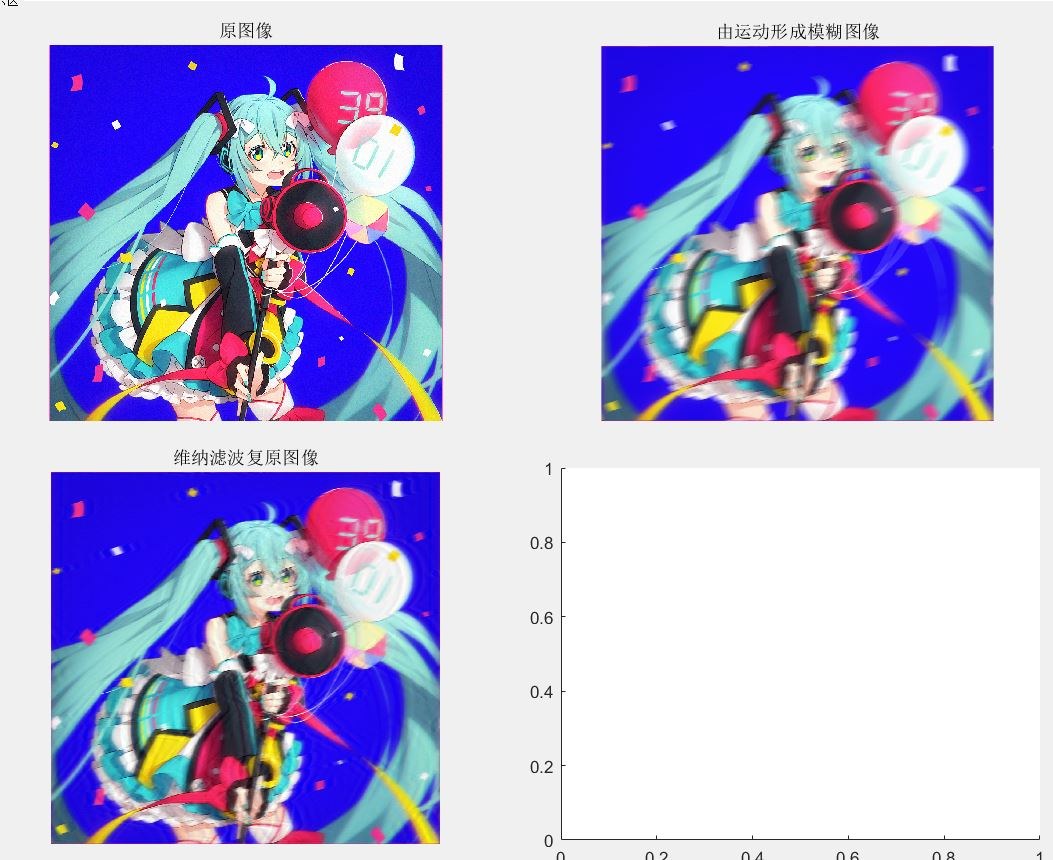（11）square膨胀

%用square结构元素膨胀图片（用text文本框比较好）
a=str2num(get(hObject,'String'));
I=handles.I;
se1=strel('square',a);
I1=imerode(I,se1);
axes(handles.axes2);    %显示在axes2框中
imshow(I1);title('用square结构元素膨胀图片');这里输入了20的数值

（12）边缘检测（使用canny算子）

I=handles.I;
I=rgb2gray(I);
BW5=edge(I,'canny');%进行canny算子边缘检测，门限值采用默认值
axes(handles.axes2);    %显示在axes2框中
imshow(BW5,[]);title('canny算子');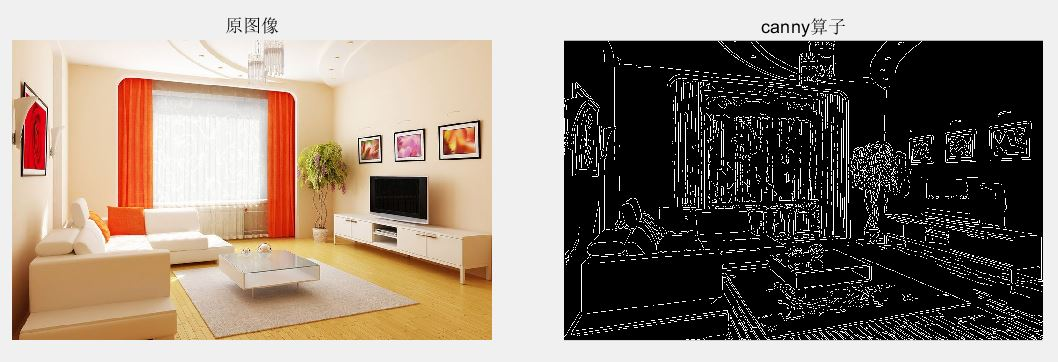附上源程序：点击打开链接

提取码：g4a5

展开全文matlab 数字图像处理 GUI 学习笔记
• 图像增强处理技术一直是图像处理领域一类非常重要的基本图像处理技术.图像增强是采用一些技 术手段 ,有选择地突出图像中感兴趣的特征或抑制图像中某些不需要的特征,使之改善图像质量、 丰富信 息量 ,加强图像判读和...
• 图像变换(傅立叶变换), 图像增强, 边缘检测, 滤波, 图像压缩等. 实验工具:MATLAB软件 课程设计时间:2008年12月 实　验　部　分 1. 图像变换 程序代码及说明 clear all N=100; f=zeros(50,50); %产生一个50*...
图像变换(傅立叶变换), 图像增强, 边缘检测, 滤波, 图像压缩等.

实验工具:MATLAB软件

课程设计时间:2008年12月

实　验　部　分

1.     图像变换

程序代码及说明

clear all

N=100;

f=zeros(50,50);          %产生一个50*50的全0数组

f(15:35,23:28)=1;

%定义图像数组，从15行到35行，23列到28列附值为1，为白色，其他区域为黑色

figure(1)                %创建窗口的图形对象，句柄为1

imshow(f,'notruesize')     %显示图像f

F=fft2(f,N,N);           %在二维傅立叶变换前把f截断或者添加0，使其成为N*N的数组

F2=fftshift(abs(F));       %把傅立叶变换的零频率部分移到频谱的中间

figure(2)

x=1:N;y=1:N;           %定义x和y的范围

mesh(x,y,F2(x,y));colormap(gray);colorbar

%绘制立体网状图，将图形对象的色度改为灰度图像，colorbar给坐标轴添加色彩条

%构建一个类似于figure(1)的矩形函数

N=200;

f=zeros(100,100);

f(30:70,45:55)=1;

%定义图像数组，从30行到70行，45列到55列附值为1，为白色，其他区域为黑色

imshow(f,'notruesize');

%然后对f进行二维快速傅立叶变换：以下列出你自己编写的代码…

N=200;

f=zeros(100,100);

f(30:70,45:55)=1;

imshow(f,'notruesize');

title('原始图像');

F=fft2(f,N,N);                 %对图像f进行二维快速傅立叶变换

grid on                       %打开网格线

axis on                       %打开坐标轴

imshow(F,[-1,5],'notruesize');     %显示傅立叶变换后的图像，图像数据的值域为[-1,5]

x=1:N;y=1:N;

title('二维快速傅立叶变换后的图像');

mesh(abs(F));                 %绘制F的频谱图

title('傅立叶变换后的频谱图');

%然后对上述二维快速傅立叶变换提高分辨率：

要提高二维快速傅立叶变换的分辨率，在采样率一定的情况下，增大采

样点数N即可。对应的频谱图见测试结果。

N=300;

f=zeros(100,100);

f(30:70,45:55)=1;

figure(1)

imshow(f,'notruesize');

title('原始图像');

F=fft2(f,N,N);

axis on

figure(2)

imshow(F,[-1,5],'notruesize');

title('二维快速傅立叶变换后的图像');

x=1:N;y=1:N;

figure(3)

mesh(abs(F));

title('傅立叶变换后的频谱图');

N=400;

f=zeros(100,100);

f(30:70,45:55)=1;

figure(1)

imshow(f,'notruesize');

title('原始图像');

F=fft2(f,N,N);

axis on

figure(2)

imshow(F,[-1,5],'notruesize');

title('二维快速傅立叶变换后的图像');

x=1:N;y=1:N;

figure(3)

mesh(abs(F));

title('傅立叶变换后的频谱图');

�系数移动（使用函数fftshift）

N=200;

f=zeros(100,100);

f(30:70,45:55)=1;

figure(1)

imshow(f,'notruesize');

title('原始图像');

F=fft2(f,N,N);

axis on

figure(2)

imshow(F,[-1,5],'notruesize');

title('二维快速傅立叶变换后的图像');

x=1:N;y=1:N;

figure(3)

mesh(fftshift(abs(F)));

%把零频率部分移到频谱的中间

title('傅立叶变换后的频谱图');

%滤波器频率响应

x=1:N;y=1:N;

mesh(x,y,F2(x,y));colormap(gray);colorbar

%绘制立体网状图，将图形对象的色度改为灰度图像，colorbar给坐标轴添加色彩条

测试结果图：

1》对f进行二维快速傅立叶变换

（请自己运行查看）

2》对上述二维快速傅立叶变换提高分辨率

N=300时：                         N=400时：

N=800时：                              N=1000时：

（请自己运行查看）

从傅立叶变换的频谱图中可以看出，提高分辨率以后，其边缘更加平滑，锯齿状明显减弱。但其傅立叶变换后的图像没有明显改变。

3》DC系数移动

其系数移动以后，频谱分量都集中到了频谱的中间。

4》滤波器频率响应

（请自己运行查看）

2. 图像增强

图像增强是指按特定的需要突出一幅图像中的某些信息，同时，消弱或去除某些不需要的信息的处理方法。其主要目的是使处理后的图像对某些特定的应用比原来的图像更加有效。图像增强技术主要包含直方图修改处理、图像平滑化处理、图像尖锐化处理、和彩色处理技术等。图像增强有图像对比度增强、亮度增强，轮廓增强等等。

下面利用直方图统计算法对灰度图像进行增强：

程序代码：

subplot(121)

imshow(I);

title('原始图像');

subplot(122)

imhist(I,64)

%绘制图像的直方图，n=64为灰度图像灰度级，若I为灰度图像，默认n=256；

若I为二值图像，默认n=2。

title('图像的直方图');

（请自己运行查看）

n=256时：

（请自己运行查看）

下面利用直方图均衡化增强图像的对比度：

J=histeq(I);

%将灰度图像转换成具有64（默认）个离散灰度级的灰度图像

imshow(I)

title('原始图像')

figure,imshow(J)

title('直方图均衡化后的图像')

figure(1)

subplot(121);imhist(I,64)

title('原始图像的直方图')

subplot(122);imhist(J,64)

title('均衡化的直方图')

（请自己运行查看）

分析：从上图中可以看出，用直方图均衡化后，图像的直方图的灰度间隔被拉大了，均衡化的图像的一些细节显示了出来，这有利于图像的分析和识别。直方图均衡化就是通过变换函数histeq将原图的直方图调整为具有“平坦”倾向的直方图，然后用均衡直方图校正图像。

下面利用直方图规定化对图像进行增强：

figure,imshow(I);

title('原始图像');

hgram=50:2:250;       %规定化函数

J=histeq(I,hgram);

figure,imshow(J);

title('直方图规定化后的图像');

figure,imhist(I,64);

title('原始图像的直方图');

figure,imhist(J,64);

title('直方图规定化后的直方图');

运行结果：

（请自己运行查看）

变换灰度间隔后的图像和直方图：

hgram=50:1:250;                    hgram=50:5:250;

3. 图像重建

图像重建的最典型的应用是医学上的计算机断层摄影技术（CT技术）。它用于人体头部、腹部等内部器官的无损伤诊断，其基本方法就是根据人体截面投影，经过计算机处理来重建截面图像。在人体中把需要扫描的部分取出一定厚度的断层面，再把断层面分成许多小的方块。当一束较窄的射线通过每个方块后强度就有一定程度的衰减，衰减的量由此方块的分子构成和组织密度决定。如果通过各种角度重复上述过程以获得一系列强度分布曲线，就有可能从这些数据中计算每一方块的衰减量。这样就能够重建断层或三维图像。

目前提出的图像重建方法有以下五种：

（1）联立方程法（也称矩阵法）；

（2）逆投影法；

（3）付立叶变换法；

（4）滤波──逆投影法（也称卷积法）；

（5）逐次逼近法。

滤波──逆投影法是当前用得较多的一种图像重建方法，在当代射线CT系统中几乎都用这种方法构成系统。它的特点是精度高，能快速实现。

程序代码：

P=phantom(256);   %用phantom函数产生Sheep-Logan的大脑图，n为图像p中的行列数，默认为256

imshow(P)

title('原始图像')

%以下为三种不同角度的投影模式

figure,imagesc(theta3,xp,R3);colormap(hot);colorbar;

xlabel('\theta');ylabel('x\prime');     %定义坐标轴

figure,imshow(I1)

figure,imshow(I2)

figure,imshow(I3)

运行结果图：

（请自己运行查看）

由上面重建的图像中可以看出，只用18个投影来重建图像效果很差，而36个投影来重建的图像要好的多，90个投影来重建的图像质量更好，失真也很小，由于R1重建图像的投影太少，所以存在许多虚假点，重建的效果与投影数目相关，投影数目越多图像重建的效果越好，所以要提高重建图像的质量，就需要增加投影角度的数目。

除此之外，还可以在Fan-Beam变换数据中用ifaanbeam函数重建图像。

4. 滤波

4.1 目的

运用中值滤波克服线性滤波器所带来的图像细节模糊。

4.2 使用设备

PC兼容机一台，操作系统为Windows2000（或Windows98，WindowsXP，以下默认为Windows2000）

4.3 使用滤波对图像进行增强

4.3.1 线性滤波（邻域平均）

线性低通滤波器最常用的是线性平滑滤波器，这种滤波器的所有系数都是正的，也称邻域平均。邻域平均减弱或消除了傅立叶变换的高频分量，对噪声的消除有所增强，但是由于平均而使图像变得更为模糊，细节的锐化程度逐渐减弱。

下面使用不同的平滑模板对图像进行滤波：（二维线性滤波fliter2）

程序代码：

imshow(I)

title('原始图像')

J=imnoise(I,'salt & pepper');    %添加盐椒噪声，噪声密度为默认值0.05

figure,imshow(J)

title('添加盐椒噪声后的图像')

K1=filter2(fspecial('average',3),J)/255;   %应用3×3邻域窗口法

figure,imshow(K1)

title('3×3窗的邻域平均滤波图像')

K2=filter2(fspecial('average',7),J)/255;   %应用7×7邻域窗口法

figure,imshow(K2)

title('7×7窗的邻域平均滤波图像')

K3=filter2(fspecial('average',9),J)/255;   %应用9×9邻域窗口法

figure,imshow(K3)

title('9×9窗的邻域平均滤波图像')

K4=filter2(fspecial('average',11),J)/255;   %应用11×11邻域窗口法

figure,imshow(K4)

title('11×11窗的邻域平均滤波图像')

运行结果图：

（请自己运行查看）

4.3.2 中值滤波

中值滤波可以保留目标边缘，这是中值滤波器相对于均值滤波器的最大优势。中值滤波具有去噪的性能，可以消除孤立的噪声点，可以用来减弱随机干扰和脉冲干扰，但是边缘不模糊。

程序代码：

imshow(I)

title('原始图像')

J=imnoise(I,'salt & pepper',0.02);  %添加盐椒噪声，噪声密度为0.02

figure,imshow(J)

title('添加盐椒噪声后的图像')

K1=medfilt2(J);               %在默认的3×3的邻域窗中进行中值滤波

figure,imshow(K1)

title('默认的3×3的邻域窗的中值滤波图像')

K2=medfilt2(J,[5 5]);           %在5×5的邻域窗中进行中值滤波

figure,imshow(K2)

title('5×5的邻域窗的中值滤波图像')

运行结果图：

（请自己运行查看）

从上可见，中值滤波的效果要比邻域平均的低通滤波效果好，中值滤波以后的图像的轮廓比较清晰，而且使用较小的模板得到的视觉效果反而好一些。

4.3.3 锐化滤波

图像锐化处理的目的是使模糊图像变得清晰，锐化滤波器减弱或消除了傅立叶空间的低频分量，保留高频分量，从而加强了图像的轮廓，使图像看起来比较清晰。

下面应用Laplacian算子对图像进行锐化处理：

Laplacian算子是线性二次微分算子，其格式为：h = fspecial('laplacian', alpha)，返回一个3×3的滤波器来近似二维Laplacian算子的形状，参数alpha决定了Laplacian算子的形状，alpha的取值范围为0.0~1.0，默认的值为0.2。

程序代码：

%应用Laplacian算子对图像进行锐化

imshow(I)

title('原始图像')

H=fspecial('laplacian');

%应用laplacian算子滤波锐化图像

laplacianH=filter2(H,I);

figure,imshow(laplacianH)

title('laplacian算子锐化后的图像')

运行结果图：

（请自己运行查看）

分析：由图可以看出，应用了Laplacian算子对图像锐化以后，将图像区域的边缘轮廓勾划了出来，因此Laplacian算子对于边缘检测也具有很好的功效。

5. 边缘检测

下面利用sobel算子对图像进行边缘检测：

使用edge函数实现图像的边缘检测，其调用格式为：

BW=edge(I,'sobel',thresh,direction)   根据指定的敏感阈值thresh用Sobel算子对图像进行边缘检测，edge函数忽略了所有小于阈值的边缘，如果没有指定阈值thresh或为空，函数自动选择参数值，direction指定Sobel算子边缘检测的方向，其参数值为'horizontal'，'vertical'或'both'（默认）。

程序代码：

imshow(I)

title('原始图像')

BW=edge(I,'sobel');

%以自动域值选择法对图像进行Sobel算子边缘检测

figure,imshow(BW);

title('自动域值的Sobel算子边缘检测')

[BW,thresh]=edge(I,'sobel');

%返回当前Sobel算子边缘检测的阈值

disp('sobel算子自动选择的阈值为：')

disp(thresh)

BW1=edge(I,'sobel',0.02,'horizontal');

%以域值为0.02水平方向对图像进行Sobel算子边缘检测

figure,imshow(BW1)

title('域值为0.02的水平方向的sobel算子检测')

BW2=edge(I,'sobel',0.02,'vertical');

%以域值为0.02垂直方向对图像进行Sobel算子边缘检测

figure,imshow(BW2)

title('域值为0.02的垂直方向的sobel算子检测')

BW3=edge(I,'sobel',0.05,'horizontal');

%以域值为0.05水平方向对图像进行Sobel算子边缘检测

figure,imshow(BW3)

title('域值为0.05的水平方向的sobel算子检测')

BW4=edge(I,'sobel',0.05,'vertical');

%以域值为0.05垂直方向对图像进行Sobel算子边缘检测

figure,imshow(BW4)

title('域值为0.05的垂直方向的sobel算子检测')

测试结果图：

（请自己运行查看）

sobel算子自动选择的阈值为：0.1433

由图可以看出，在采用水平和垂直方向的Sobel算子对图像进行边缘检测时，分别对应的水平和垂直方向上的边缘有较强的响应，阈值越小，检测的图像的边缘细节数越多，而增大阈值时，有些轮廓则未能检测出。

问题与思考：

根据Prewitt算子的定义设计实现用Prewitt算子进行图像的边缘检测。

其用法和Sobel算子类似。其调用格式为：

BW=edge(I,'prewitt',thresh,direction) 根据指定的敏感阈值thresh用Prewitt算子对图像进行边缘检测。

程序代码：

imshow(I)

title('原始图像')

BW=edge(I,'prewitt');

%以自动域值选择法对图像进行Prewitt算子边缘检测

figure,imshow(BW);

title('自动域值的prewitt算子边缘检测')

[BW,thresh]=edge(I,'prewitt');

%返回当前Prewitt算子边缘检测的阈值

disp('prewitt算子自动选择的阈值为：')

disp(thresh)

BW1=edge(I,'prewitt',0.02,'horizontal');

%以域值为0.02水平方向对图像进行Prewitt算子边缘检测

figure,imshow(BW1)

title('域值为0.02的水平方向的prewitt算子检测')

BW2=edge(I,'prewitt',0.02,'vertical');

%以域值为0.02垂直方向对图像进行Prewitt算子边缘检测

figure,imshow(BW2)

title('域值为0.02的垂直方向的prewitt算子检测')

BW3=edge(I,'prewitt',0.05,'horizontal');

%以域值为0.05水平方向对图像进行Prewitt算子边缘检测

figure,imshow(BW3)

title('域值为0.05的水平方向的prewitt算子检测')

BW4=edge(I,'prewitt',0.05,'vertical');

%以域值为0.05垂直方向对图像进行Prewitt算子边缘检测

figure,imshow(BW4)

title('域值为0.05的垂直方向的prewitt算子检测')

测试结果图：

（请自己运行查看）

prewitt算子自动选择的阈值为：0.1399

6. 图像压缩

图像压缩就是就是通过去除这些数据冗余来减少表示数据所需的比特数，去除多余数据。以数学的观点来看，这一过程实际上就是将二维像素阵列变换为一个在统计上无关联的数据集合。

图像压缩是指以较少的比特有损或无损地表示原来的像素矩阵的技术，也称图像编码。

图像数据之所以能被压缩，就是因为数据中存在着冗余。图像数据的冗余主要表现为：图像中相邻像素间的相关性引起的空间冗余；图像序列中不同帧之间存在相关性引起的时间冗余；不同彩色平面或频谱带的相关性引起的频谱冗余。

从压缩编码算法原理上可以分类为：

(1)无损压缩编码种类：哈夫曼编码、算术编码、行程编码、Lempel zev编码

(2)有损压缩编码种类：

预测编码：DPCM，运动补偿

频率域方法：正交变换编码(如DCT)，子带编码

空间域方法：统计分块编码

模型方法：分形编码，模型基编码

基于重要性：滤波，子采样，比特分配，矢量量化

(3)混合编码 ?JBIG，H261，JPEG，MPEG等技术标准

利用余弦变换实现图像压缩：

DCT先将整体图像分成N×N像素块（一般N=8 ，即64个像素块），再对N×N块像素逐一进行DCT变换。由于大多数图像高频分量较小，相应于图像高频成分的失真不太敏感，可以用更粗的量化，在保证所要求的图质下，舍弃某些次要信息。

程序代码：

imshow(I);

title('原始图像')

disp('原始图像大小：')

whos('I')

I=im2double(I);

%图像类型存储转换，将图像矩阵转换成双精度类型

T=dctmtx(8);

%离散余弦变换矩阵

B=blkproc(I,[8 8],'P1*x*P2',T,T');

mask=[1 1 1 1 0 0 0 0

1 1 1 0 0 0 0 0

1 1 0 0 0 0 0 0

1 0 0 0 0 0 0 0

0 0 0 0 0 0 0 0

0 0 0 0 0 0 0 0

0 0 0 0 0 0 0 0

0 0 0 0 0 0 0 0];

I2=blkproc(B2,[8 8],'P1*x*P2',T',T);

figure,imshow(I2);

title('压缩后的图像')

disp('压缩图像的大小：')

whos('I2')

运行结果：

（请自己运行查看）

原始图像大小：

Name        Size              Bytes  Class    Attributes

I         256x256            65536  uint8

压缩图像的大小：

Name        Size              Bytes  Class     Attributes

I2        256x256            524288  double

分析：由运行结果可以看出，经过DCT变换以后图像的大小几乎没有改变，我们知道DCT是一种空间变换，DCT变换的最大特点是对于一般的图像都能够将像块的能量集中于少数低频DCT系数上，这样就可能只编码和传输少数系数而不严重影响图像质量。DCT不能直接对图像产生压缩作用，但对图像的能量具有很好的集中效果，为压缩打下了基础。例如：一帧图像内容以不同的亮度和色度像素分布体现出来，而这些像素的分布依图像内容而变，毫无规律可言。但是通过离散余弦变换(DCT)，像素分布就有了规律。代表低频成份的量分布于左上角，而越高频率成份越向右下角分布。然后根据人眼视觉特性，去掉一些不影响图像基本内容的细节(高频分量)，从而达到压缩码率的目的。

利用小波变换实现图像压缩：

程序代码：

clear all

imshow(I);                   %显示图像

title('原始图像')

disp('原始图像I的大小:');

whos('I')

I=im2double(I);

[c,s]=wavedec2(I,2,'bior3.7');     %对图像用小波进行层分解

cal=appcoef2(c,s,'bior3.7',1);     %提取小波分解结构中的一层的低频系数和高频系数

ch1=detcoef2('h',c,s,1);          %提取二维水平方向细节系数

cv1=detcoef2('v',c,s,1);          %提取二维垂直方向细节系数

cd1=detcoef2('d',c,s,1);          %提取二维对角线方向细节系数

ca1=appcoef2(c,s,'bior3.7',1);     %保留小波分解第一层低频信息

ca1=wcodemat(ca1,440,'mat',0);   %首先对第一层信息进行量化编码

ca1=0.5*ca1;                  %改变图像高度

figure,image(ca1);              %显示压缩后的图象

title('第一次压缩后的图像')

disp('第一次压缩图像的大小为：')

whos('ca1')

ca2=appcoef2(c,s,'bior3.7',2);     %保留小波分解第二层低频信息进行压缩

ca2=wcodemat(ca2,440,'mat',0);   %首先对第二层信息进行量化编码

ca2=0.25*ca2;                 %改变图像高度

figure,image(ca2);              %显示压缩后的图象

title('第二次压缩后的图像')

disp('第二次压缩图像的大小为：')

whos('ca2')

运行结果：

（请自己运行查看）

原始图像I的大小:

Name      Size                   Bytes  Class

I       256x256                  65536  uint8 array

Grand total is 65536 elements using 65536 bytes

第一次压缩图像的大小为：

Name      Size                   Bytes  Class

ca1     135x135                 145800  double array

Grand total is 18225 elements using 145800 bytes

第二次压缩图像的大小为：

Name      Size                   Bytes  Class

ca2      75x75                   45000  double array

Grand total is 5625 elements using 45000 bytes

分析：利用小波变换实现图像压缩，是利用小波分解图像的高频部分，只保留低频部分，从图中可以看出，第一次压缩是提取图像中小波分解的第一层低频信息，此时压缩效果较好，第二次压缩是提取第二层的低频部分，其压缩效果远不如第一次压

展开全文• 最近一直在讲matlab图像处理，其目的是让大家后边更好的对比与fpga处理的过程，matlab图像处理相对简单，只需要调用相应的函数。fpga需要对每个函数的处理过程以及每个步骤都要了解。 后续我还会讲到matlab的...
基于matlab的图像中心差分处理

最近一直在讲matlab的图像处理，其目的是让大家后边更好的对比与fpga处理的过程，matlab图像处理相对简单，只需要调用相应的函数。fpga需要对每个函数的处理过程以及每个步骤都要了解。

后续我还会讲到matlab的人脸识别算法的实现，也将尝试fpga的人脸识别，希望大家鼓励。

在处理图像的时候，特别是处理视频流图像的时候，往往会用到图像差分的方法。顾名思义，图像差分，就是把两幅图像的对应像素值相减，以削弱图像的相似部分，突出显示图像的变化部分。例如，差分图像往往能够检测出运动目标的轮廓，能够提取出闪烁导管的轨迹等等。

中心差分源码：

figure; imshow(I);

forward_dx = mipforwarddiff(I,'dx'); figure, imshow(forward_dx);

forward_dy = mipforwarddiff(I,'dy'); figure, imshow(forward_dy);

central_dx = mipcentraldiff(I,'dx'); figure, imshow(central_dx);

central_dy = mipcentraldiff(I,'dy'); figure, imshow(central_dy);

function dimg = mipcentraldiff(img,direction)

% MIPCENTRALDIFF     Finite difference calculations

%

%   DIMG = MIPCENTRALDIFF(IMG,DIRECTION)

%

%  Calculates the central-difference for a given direction

%  IMG       : input image

%  DIRECTION : 'dx' or 'dy'

%  DIMG      : resultant image

%

[row,col] = size(img);

dimg = zeros(row,col);

switch (direction)

case 'dx',

dimg(:,2:col-1) =(img(:,3:col)-img(:,1:col-2))/2;

case 'dy',

dimg(2:row-1,:) =(img(3:row,:)-img(1:row-2,:))/2;

otherwise,

disp('Direction is unknown');

end

dimg = dimg(2:end-1,2:end-1);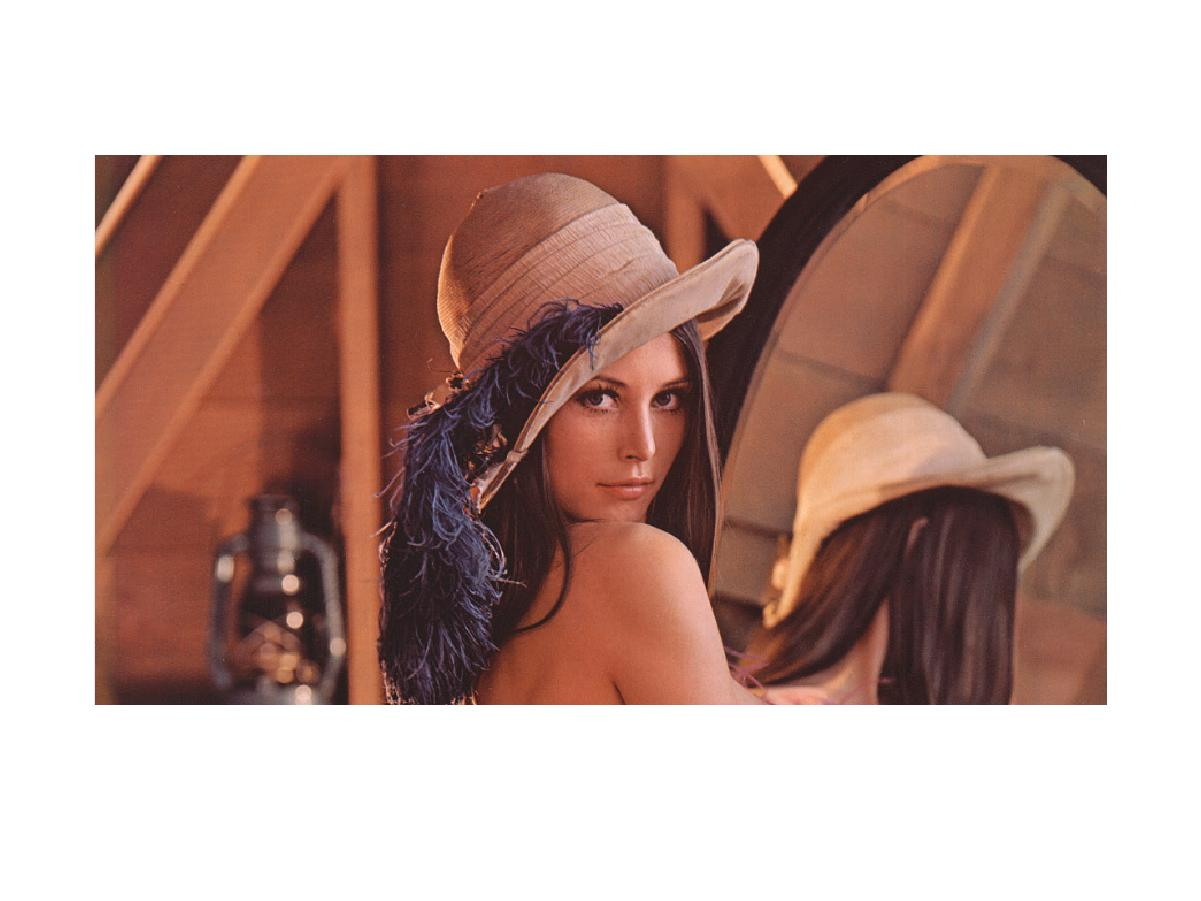原图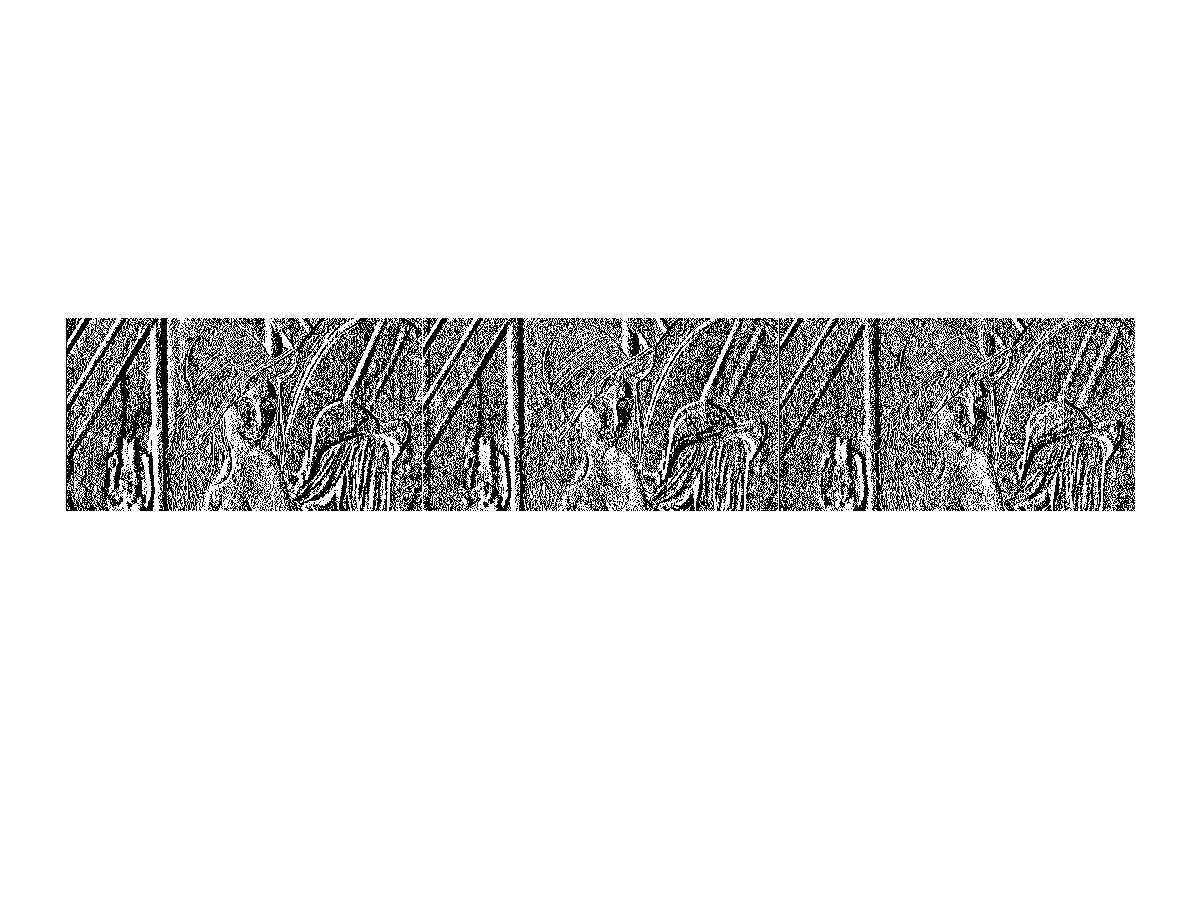x方向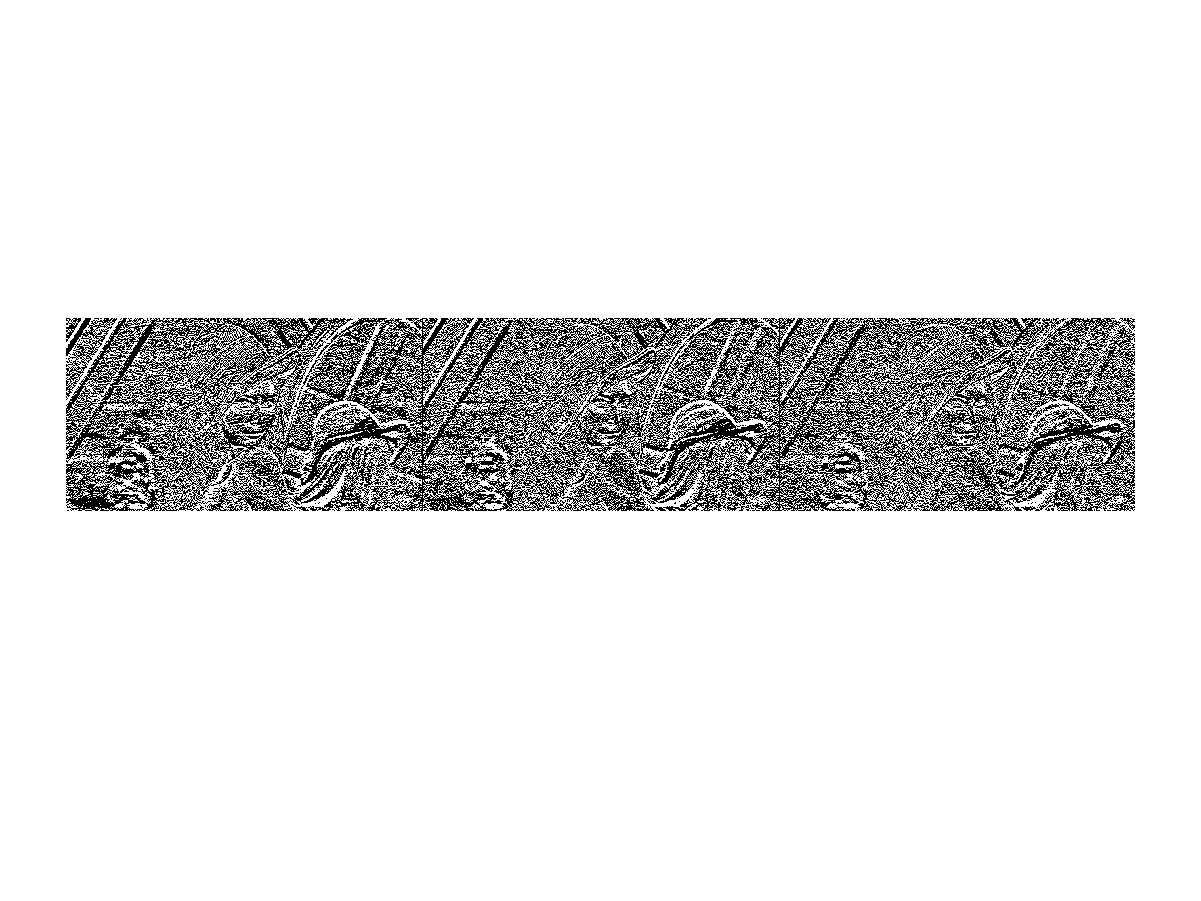y方向

FPGA自习学院将不断更新和总结FPGA相关学习资料，书籍，实例工程和视频。

欢迎大家加入FPGA自习学院，一起学习一起成长。最后欢迎大家关注我的微信公众号，我将陆续提供更多的FPGA基础教程展开全文matlab 图像处理
• 平滑滤波——matlab图像处理 平滑滤波的目的是消除或尽量减少噪声，改善图像的质量。假设加性噪声是随机独立分布，这样利用图像像素领域的平均或加权平均即可有效地抑制噪声干扰。从信号分析的观点来看，图像平滑...
平滑滤波——matlab图像处理
平滑滤波的目的是消除或尽量减少噪声，改善图像的质量。假设加性噪声是随机独立分布，这样利用图像像素领域的平均或加权平均即可有效地抑制噪声干扰。从信号分析的观点来看，图像平滑本质上是低能滤波，信号的低频部分可通过，高频的噪声信号被阻截。但由于图像边缘也处于高频部分，这样往往带来另一个问题：在对图像进行平滑处理时，往往对图像的细化造成一定程度的损坏。
领域运算可用领域与模版的卷积得到，这极大地方便了计算。
MATLAB中提供的imfliter函数用于实现图像的平滑处理，其调用格式如下。
B=imfliter（A，H）：使用多维滤波器H对图像A进行滤波（平滑）。参数A可以是任意维的二值或非奇异数值型矩阵。参数H为矩阵，表示滤波器。H常由函数fspecial输出得到。返回值B与A的维数相同。
B=imfliter（A,H，optional1，optional2，…）

参数类型
说明

X
输入图像的外边界通过X来扩展，X默认值为0

symmetric
输入图像的外部边界通过镜像反射其内部边界来扩展

circular
输入图像的别界通过假设输入图像为周期函数来扩展

relicate
输入图像的外部别界通过复制内部别界的值来扩展

same
输入和输出图像大小相等，默认操作

full
输出图像比输入图像大

corr
使用相关进行滤波（平滑）

conv
使用卷积进行滤波（平滑）

matlab中提供的fspecial函数用于创建二维滤波器：
h=fspecial（type）：
type可以是：average，disk，gaussian，laplacian，log，motion，prewitt，sobel，unsharp。
h=fspecial（type，parameters）：创建指定类型和指定参数的二维滤波器h。参数parameters为与滤波器有关的参数。
clear all;
subplot(2,2,1);imshow(I);
xlabel('(a)原始图像');
H = fspecial('motion',20,45);
MotionBlur = imfilter(I,H,'replicate');
subplot(2,2,2);imshow(MotionBlur);
xlabel('(b)运动模糊图像');
H=fspecial('disk',10);
blurred = imfilter(I,H,'replicate');
subplot(2,2,3);imshow(blurred);
xlabel('(c)模糊图像');
H=fspecial('unsharp');
sharpened = imfilter(I,H,'replicate');
subplot(2,2,4);imshow(sharpened);
xlabel('(d)锐化图像');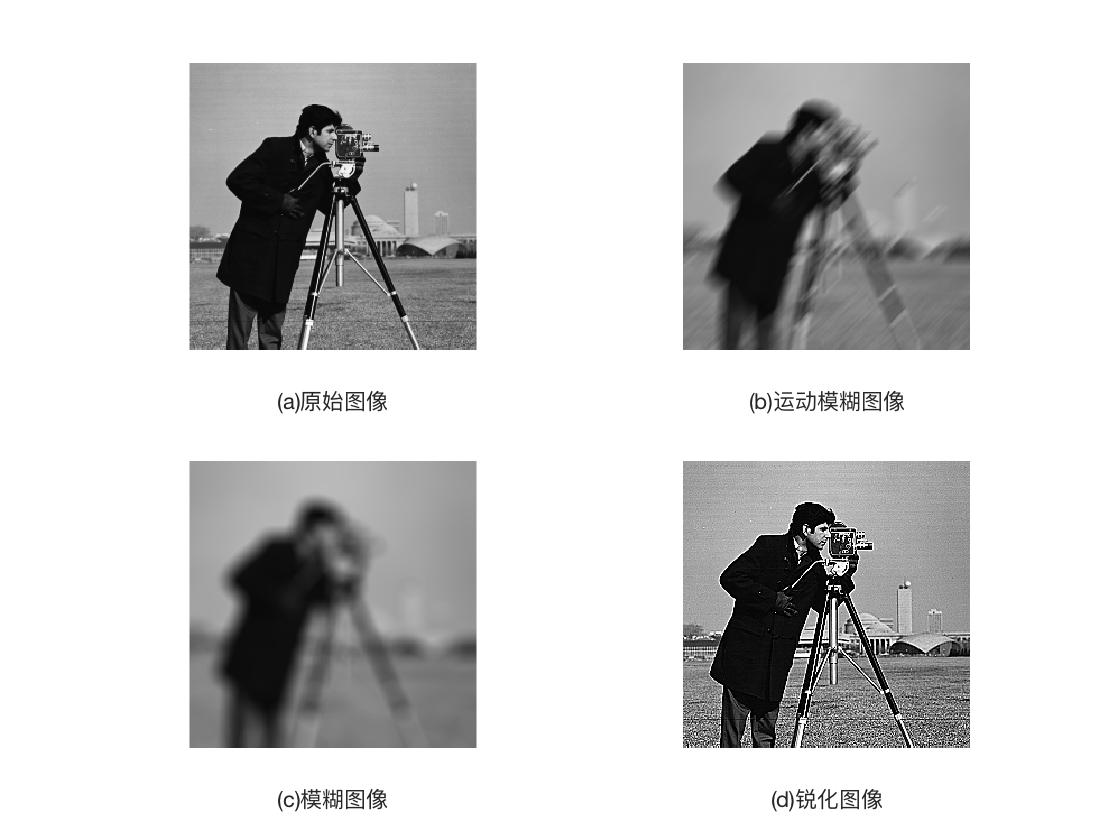对含有高斯噪声的图像进行平滑处理。
clear all;
Inoised = imnoise(I,'gaussian',0.1,0.005);%对图像进行高斯噪声加噪
%制定卷积核
h=ones(3,3)/5;
h(1,1) = 0;
h(1,3) = 0;
h(3,1) = 0;
h(1,3) = 0;
%平滑运算
I2=imfilter(Inoised,h);
subplot(1,3,1);imshow(I);
xlabel('(a)原始图像');
subplot(1,3,2);imshow(Inoised);
xlabel('(b)带噪声图像');
subplot(1,3,3);imshow(I2);
xlabel('(c)平滑后图像');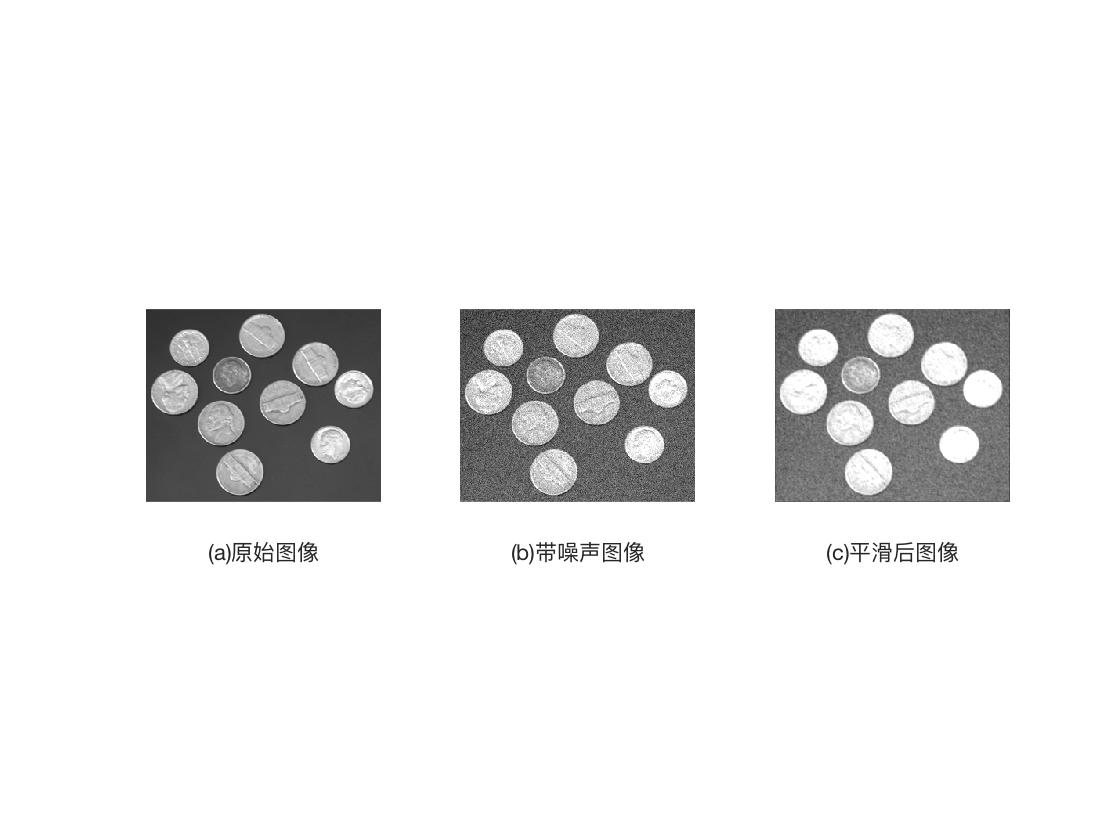有问题还请多多指教。刚刚入门。谢谢各位大牛。


展开全文平滑滤波 Matlab
• 图像在形成、记录、处理和传输过程中，由于成像 系统、记录设备、传输介质和处理方法的不完善， 会导致图像质量下降。这一过程称为图像的退化。 引起图像质量下降的客观因素 − 成像系统的像差、畸变、带宽有限等...
• 原文地址：基于MATLAB图像处理程序（全文转载）作者：星夜图像变换(傅立叶变换), 图像增强, 边缘检测, 滤波, 图像压缩等. 实验工具:MATLAB软件 课程设计时间:2008年12月 实　验　部　分 1.   图像变换 程序代码...
• MATLAB图像与视频处理实用案例详解》详细讲解了25个MATLAB图像与视频处理实用案例（含可运行程序），涉及雾霾去噪、答题卡自动阅卷、肺部图像分割、小波数字水印、图像检索、人脸二维码识别、车牌定位及识别、...MATLAB
• 一、图像处理预备知识1、图像处理(image processing)用计算机对图像进行分析，以达到所需结果的技术。又称影像处理。图像处理一般指数字图像处理。在计算机中，按照颜色和灰度的多少可以将图像分为二值图像、灰度...
• MATLAB图像与视频处理实用案例详解》详细讲解了25个MATLAB图像与视频处理实用案例（含可运行程序），涉及雾霾去噪、答题卡自动阅卷、肺部图像分割、小波数字水印、图像检索、人脸二维码识别、车牌定位及识别、...图像
• matlab图像与视频实用案例详解pdf+源码、模糊图像复原源码加图片、运动图像点扩散函数
• 我数字图像处理的作业，内有实验报告、m文件、图像文件 包括的实验为： 1.Roberts算子、Sobel算子、Prewitt算子、拉普拉斯算子、LoG算子和Canny算子等进行边缘检测 2.手动阈值分割 3.迭代阈值和OTSU算法对图像进行二...
• 数字图像处理技术的广泛应用，其中有很多相应的处理工具，包括ps等，也可以通过matlab编程来进行数字图像的处理。
• MATLAB图像与视频处理实用案例详解》详细讲解了25个MATLAB图像与视频处理实用案例（含可运行程序），涉及雾霾去噪、答题卡自动阅卷、肺部图像分割、小波数字水印、图像检索、人脸二维码识别、车牌定位及识别、...
• 之前由于双目测距的工作，用MATLAB获取摄像头数据并实时处理。现将一些基本操作分享给大家。欢迎交流MATLAB
• 中科院期末王伟强数字图像处理大作页，用MATLAB实现了冈萨雷斯书中的大部分程序，带有可视化的界面，可以调节参数，实现的功能简要概括如下：灰度变换与图像锐化，滤波（低通，高通，中值，维纳滤波），噪声模型，...
...